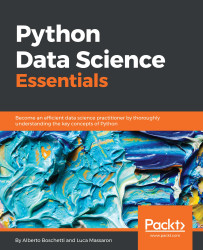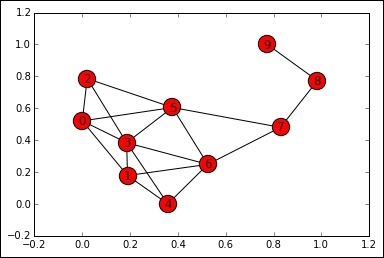•#### Python Data Science Essentials#### Overview of this book

The book starts by introducing you to setting up your essential data science toolbox. Then it will guide you across all the data munging and preprocessing phases. This will be done in a manner that explains all the core data science activities related to loading data, transforming and fixing it for analysis, as well as exploring and processing it. Finally, it will complete the overview by presenting you with the main machine learning algorithms, the graph analysis technicalities, and all the visualization instruments that can make your life easier in presenting your results. In this walkthrough, structured as a data science project, you will always be accompanied by clear code and simplified examples to help you understand the underlying mechanics and real-world datasets.
Python Data Science EssentialsCreditswww.PacktPub.comPrefaceFree Chapter
First StepsData MungingThe Data Science PipelineMachine LearningSocial Network AnalysisVisualizationIndex## Graph algorithms

To get insights from graphs, many algorithms have been developed. In this chapter, we'll use a well-known graph in `NetworkX`, the `Krackhardt Kite` graph. It is a dummy graph containing 10 nodes, and it is typically used to proof graph algorithms. Krackhardt is the creator of the structure, which has the shape of a kite. It's composed of two different zones. In the first zone (composed of nodes 0 to 6), the nodes are interlinked; in the other zone (nodes 7 to 9), they are connected as a chain:

```In: G = nx.krackhardt_kite_graph()
nx.draw_networkx(G)
plt.show()
```Let's start with connectivity. Two nodes of a graph are connected if there is at least a path (that is, a sequence of traversed nodes) between them.

### Note

Note that in a directed graph, you must follow the link's directions.

If at least a path exists, the shortest path between the two nodes is the one with the shortest collection of traversed nodes.

In `NetworkX`, checking whether a path exists between two nodes, calculating the...